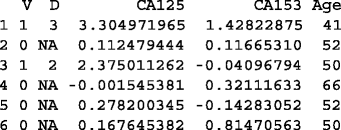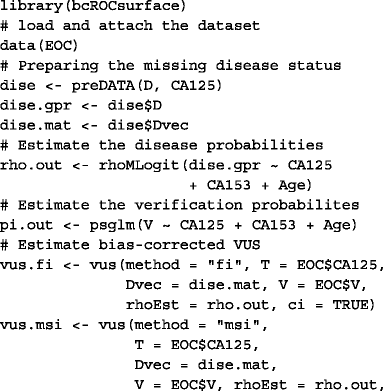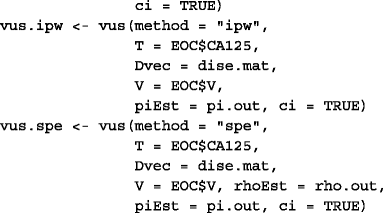# bcROCsurface: an R package for correcting verification bias in estimation of the ROC surface and its volume for continuous diagnostic tests

## Abstract

### Background

Receiver operating characteristic (ROC) surface analysis is usually employed to assess the accuracy of a medical diagnostic test when there are three ordered disease status (e.g. non-diseased, intermediate, diseased). In practice, verification bias can occur due to missingness of the true disease status and can lead to a distorted conclusion on diagnostic accuracy. In such situations, bias–corrected inference tools are required.

### Results

This paper introduce an R package, named bcROCsurface, which provides utility functions for verification bias–corrected ROC surface analysis. The shiny web application of the correction for verification bias in estimation of the ROC surface analysis is also developed.

### Conclusion

bcROCsurface may become an important tool for the statistical evaluation of three–class diagnostic markers in presence of verification bias. The R package, readme and example data are available on CRAN. The web interface enables users less familiar with R to evaluate the accuracy of diagnostic tests, and can be found at http://khanhtoduc.shinyapps.io/bcROCsurface_shiny/.

## Background

The use of diagnostic tests is becoming more and more popular in medicine, a popularity that feeds the need for assessing their accuracy. A common approach employed to this aim is receiver operating characteristic (ROC) analysis. For a three class disease status (e.g., non-diseased, intermediate and diseased), the ROC surface and the volume under ROC surface (VUS) are frequently used. The graph of ROC surface lies in the unit cube and the VUS varies from 0 to 1. More precisely, the ROC surface of a useless test is the plane of the triangle with three vertices (1,0,0), (0,1,0) and (0,0,1), whereas the ROC surface corresponding to a perfect test is the surface of the unit cube. Consequently, the value of VUS is 1/6 in case of useless tests, and 1 if the diagnostic test is perfect.

There are various methods  for estimating a ROC surface and its VUS when all subjects in the study undergo a gold standard (GS) test, a condition often referred to as full verification of subjects. In R, some packages exist for ROC surface analysis under full verification. For example, DiagTest3Grp  gives some tools for estimating VUS, ROCS  deals with the high-throughput class-skewed data and HUM  provides tools for visualizing the ROC surface.

Usually, however, only a subset of subjects is selected to undergo disease verification, due to the expensiveness and/or invasiveness of the GS test. If only the verified subjects are used for estimating the ROC surface and VUS, inference tools are biased, an effect known as verification bias. No package is available for correcting for verification bias estimators of the ROC surface and VUS. The R package bcROCsurface aims at filling this gap. It provides several new functions for bias-corrected ROC surface analysis. More precisely, it implements methods in To Duc et al. [5, 6], who proposed five bias-corrected estimators for ROC surface and VUS of a continuous diagnostic test, namely, full imputation (FI), mean score imputation (MSI), inverse probability weighting (IPW), semiparametric efficient (SPE) and K nearest-neighbor (KNN) estimators. These methods perform provided that the missing mechanism is MAR (missing at random).

## Implementation

The bcROCsurface imports various R packages (e.g., rgl, nnet, boot) and is built on Rcpp . The package is freely available to download from CRAN - a global repository of R packages http://cran.r-project.org, and provides several functions for bias–corrected inference on VUS, for constructing and plotting 3D-ROC surfaces as well as ellipsoidal confidence regions of true class fractions at a given cut-point.

The data to be elaborated should include: a variable representing the disease status (categorical variable with three classes), a variable representing the diagnostic test (continuous variable) and a variable representing the verification status (binary variable, 1 and 0 indicate verified and not verified subject, respectively). Some other auxiliary covariates (numeric variables) may also be present. Practical use of the package foresees three steps: data preparation, modeling and inference.

### Data preparation

In this step, the condition of monotone ordering of the three disease classes under study  is checked. The condition is mandatory to perform the subsequent analyses. In words, the condition assumes that subjects from class 3 have higher test results than subjects in class 2 and the latter have higher test results than subjects in class 1. Function preDATA() performs such checks, warning users in case monotone ordering is not satisfied. When satisfied, the function coerces the disease status in the numeric format (1, 2, 3) corresponding to increasing disease status. It also generates a binary matrix with three columns, corresponding to the coding of the three classes of the disease status, used as input of the main functions.

### Modeling

Correction for verification bias requires estimation of a disease and a verification model. The function psglm() obtains the verification probabilities specifying a general linear model for the verification process. In practice, the user can select among a logistic, a probit or a threshold regression model (default is logistic model). Functions rhoMLogit() and rhoKNN() estimate the disease probabilities based on a multinomial logistic regression. In particular, rhoMLogit() calls the nnet package for multinomial logistic modeling, whereas rhoKNN() uses K nearest-neighbor regression.

### Inference

Two main functions are provided: ROCs() for constructing and plotting ROC surfaces, and vus() for estimating VUS values as well as obtaining confidence intervals. Estimation methods can be flexibly selected by the argument method, among 6 options, i.e., full if the full data is available; fi for the FI estimator, msi for the MSI estimator, ipw for the IPW estimator, spe for the SPE estimator and knn for the KNN estimator in presence of partial verification (see [5, 6] for the definition of the estimators). To plot ROC surfaces and ellipsoid confidence regions, the function ROCs() employs the plotting functions of the rgl package. vus() employs some core functions, written in the C++ language and integrated in R through the Rcpp and RcppArmadillo packages. Confidence intervals of VUS values are built based on the asymptotic distribution or the bootstrap resampling process (supported by the parallel computing). In addition, this function also performs the statistical test, H0: VUS = 1/6 versus H1: VUS > 1/6. The test statistic is

$$t = \frac{\widehat{\text{VUS}} - 1/6}{\sqrt{{\widehat{\text{Var}}(\widehat{\text{VUS}})}}} \overset{.}{\sim} \mathcal{N}(0,1),$$

where $$\widehat {\text {VUS}}$$ is the estimator of VUS and $$\widehat {\text {Var}}(\widehat {\text {VUS}})$$ denotes the estimate of the variance of $$\widehat {\text {VUS}}$$.

Besides the functions described above, the package also offers other functions for estimating variances and for choosing K to compute the KNN estimate.

A Shiny web application has also been developed to provide the possibility to deploy bcROCsurface package over the web. The layout of the bcROCsurface web interface is clean and straightforward (Fig. 1). It provides the possibility to load the datasets for the analysis and to access all functions of bcROCsurface package. Here, the user loads a data file (typically,.csv,.txt or.dat file), selects a suitable option for “Separator” and “Quote” to read data correctly, then chooses the input variables, i.e. diagnostic test, disease status. If the true disease status is not missing, the user follows step 1 and 2 to get the results. Otherwise, the user clicks on the square box and selects the verification status, then follows step 1, 2 and 3 to implement the bias-corrected ROC surface analysis.

## Results and discussion

In the following example, the package is employed to evaluate the accuracy of ovarian cancer-related tumor marker CA125 (cancer antigen 125), which is a highly glycosylated sialomucin that is expressed on epithelial cell surface, especially on ovarian cancer cells. The dataset, available in the package, is described in detail in . In what follows, CA125 will be the diagnostic test of interest and CA153 and Age will be two auxiliary covariates. Three classes of cancer are considered, i.e., benign (1), early stage (2) and late stage (3). The first six lines of the dataset are shown below (V denotes verification status and D disease status).As mentioned above, in the first step, the application of preDATA() is needed to ensure that the package can be employed. In the second step, to produce estimators FI, MSI, IPW and SPE, the functions rhoMLogit() and psglm() are called to fit the disease model and verification model. Finally, vus() is used to obtain the bias-corrected estimates of VUS for marker CA125 and the values of the statistic t for testing H0: VUS = 1/6 vs. H1: VUS > 1/6. Results were produced using R code below.Table 1 shows the four bias-corrected estimates of VUS, associated standard error and approximate 95% confidence intervals built with and without logit transformation. Table 2 shows the values of t-stat as well as p-values for testing H0: VUS = 1/6 vs. H1: VUS > 1/6. Statistical interpretation of results in Table 1 is given in , Section 5.1. Being this an artificial example, i.e., an example in which missingness of the disease has been artificially created according to the MAR assumption, the above mentioned section explains how to evaluate usefulness of bias correction and why SPE and IPW can be considered good estimates in this case.

The shiny web application is also easy to use. By using it, the four bias-corrected ROC surfaces of biomarker CA125, described above, can be easily obtained and are shown in Fig. 2.

From a computational point of view, the demand of bcROCsurface is essentially associated to estimation of variances of VUS (see ). To establish the computation time of the functions asyVarVUS() and vus(), a simulation study is performed. The disease status is generated as a trinomial random vector (D 1,D 2,D 3), such that D k is a Bernoulli random variable with mean θ k , k=1,2,3. Setting θ 1=0.4,θ 2=0.35 and θ 3=0.25. A diagnostic test T and an auxiliary covariate A are generated from the following conditional models

$$T,A |D_{k} \sim \mathcal{N}_{2} \left(k \left({2 \atop 1}\right), \left(\begin{array}{cc} 1.75 & 0.1 \\ 0.1 & 2.5 \end{array}\right) \right), \ \ \quad k = 1,2,3.$$

The verification status V is simulated by using the following model

$$\text{logit}\left\{\Pr(V = 1|T,A)\right\} = 1 - 2.2 T + 4 A.$$

In this simulation, the SPE estimator is employed (being the most computationally demanding) and the sample size varies from 200 to 2000. The computation is replicated 100 times and is performed on a PC Intel(R) Core(TM) i7-2720QM CPU, 2.2 GHz, 8.00 GB RAM. The average elapsed times (in seconds) of the functions vus() and of asyVarVUS() are shown in Fig. 3.

## Conclusions

The paper presents the R package bcROCsurface, that provides routines to construct the ROC surface and estimate the VUS for continuous diagnostic tests when disease status is missing at random. The shiny web interface is straightforward to use and is therefore accessible to users less familiar with the programming language R.

## Availability and requirements

The bcROCsurface package is available on CRAN (http://CRAN.R-project.org/package=bcROCsurface), which is compatible with any operating system supporting R program. The license is GPL-2 | GPL-3. The Shiny web application is freely available to all users from http://khanhtoduc.shinyapps.io/bcROCsurface_shiny/.

## Abbreviations

FI:

Full imputation

IPW:

Inverse probability weighting

KNN:

K nearest-neighbor

MAR:

Missing at random

MSI:

Mean score imputation

ROC:

SPE:

Semi-parametric efficient

VUS:

Volume under ROC surface

## References

1. Nakas CT. Developments in ROC surface analysis and assessment of diagnostic markers in three-class classification problems. REVSTAT–Stat J. 2014; 12:43–65.

2. Luo J, Chengjie X. DiagTest3Grp: an R package for analyzing diagnostic tests with three ordinal groups. J Stat Softw. 2012; 53(3):1–24.

3. Yu T. ROCS: receiver operating characteristic surface for class-skewed high-throughput data. PLOS ONE. 2012; 7:7:e40598.

4. Novoselova N, Beffa CD, Wang J, Li J, Pessler F. HUM calculator and HUM package for R: easy-to-use software tools for multicategory receiver operating characteristic analysis. Bioinformatics. 2014; 30:1635–1636.

5. To Duc K, Chiogna M, Adimari G. Bias-corrected methods for estimating the receiver operating characteristic surface of continuous diagnostic tests. Electron J Stat. 2016; 10:3063–3113.

6. To Duc K, Chiogna M, Adimari G. Nonparametric estimation of ROC surfaces under verification bias. https://arxiv.org/abs/1604.04656. Submitted.

7. Eddelbuettel D. Seamless R and C++ integration with Rcpp. New York: Springer; 2013.

8. Nakas CT, Yiannoutsos CY. Ordered multiple-class ROC analysis with continuous measurements. Stat Med. 2004; 23:3437–49.

## Acknowledgements

The author thanks Monica Chiogna and Gianfranco Adimari from Department of Statistical Sciences, University of Padova, for their comments that helped to improve and refine the implementation.

### Funding

No funding was received for the study.

## Author information

Authors

### Contributions

KTD wrote the bcROCsurface package, performed the analysis, and wrote the manuscript.

### Corresponding author

Correspondence to Khanh To Duc.

## Ethics declarations

Not applicable.

Not applicable.

### Competing interests

The author has no competing interests.

### Publisher’s Note

Springer Nature remains neutral with regard to jurisdictional claims in published maps and institutional affiliations.

## Rights and permissions